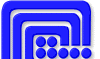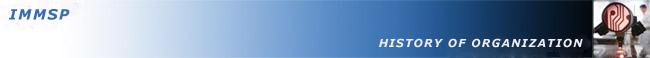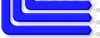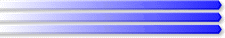ukrainian version

A study methodology of the statistical instability of physical quantities and processes is developed. The connection between the statistically unstable processes and flicker-noises was established. The method of process optimization and transformation of electromagnetic energy scheme of the current generator of industrial frequency where in comparison with existing generators, the consumption of mechanical energy was reduced while maintaining the value of electricity consumption for generating power were proposed.

Numerical and analytical methods for physical phenomena modeling under conditions of uncertainty and methods for ill conditioned problems solving were developed.

The numerical and analytical methods of physical phenomena modeling under the conditions of uncertainty and methods for solving poorly conditioned problems were developed.

The methodology of assessing the level of statistical stability of physical processes was developed. Physico-mathematical theory of hyper random phenomena was developed. The theory provides a description of real physical events, variables, processes and fields considering their peculiar limited statistical stability.

The method of correct formulation of the problem of modeling of physical phenomena in the condition of uncertainty was developed as well as the possibility of using the results obtained in solving poorly conditioned problems was explored.

Physico-mathematical theory of hyper phenomena was developed. The results are summarized in the monograph of Gorban I.I. The Statisical Stability Phenomenon (Phenomenon statistical stability). – Springer, 2016. – 362 p. – ІSВN 978-3-319-43584-8.

A new method of measuring physical quantities under conditions of statistical stability has been developed. It provides a significant increase in the accuracy of the measurement. In the interest of improving the quality of processing long signals and the accuracy of measurements and forecasting the development of events is essential to in-depth study of statistical stability as a physical phenomenon. The obtained results are summarized in the scientific monograph by Gorban I.I. Randomness and Hyper-randomness. - Springer, 2017 - 248 p.